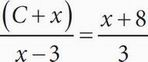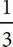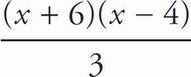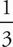# SAT Math Multiple Choice Question 92: Answer and Explanation

### Test Information

Question: 92

2. If, which of the following could be an expression of C in terms of x ?

• A. 3(1 + x)
• B. x2 + 2x - 24
• C.(x + 6)(x - 4)
• D.(x - 3)(x + 8)

C Cross-multiply to get 3(C + x) = (x - 3)(x + 8). Expand the right side of the equation to get 3(C + x) = x2 + 5x - 24. Distribute the 3 to get 3C + 3x = x2 + 5x - 24. Subtract 3x from both sides of the equation to get 3C = x2 + 2x - 24. Factor the right side of the equation to get 3C = (x + 6)(x - 4). Divide both sides by 3 to get C ==(x + 6)(x - 4). The correct answer is (C).